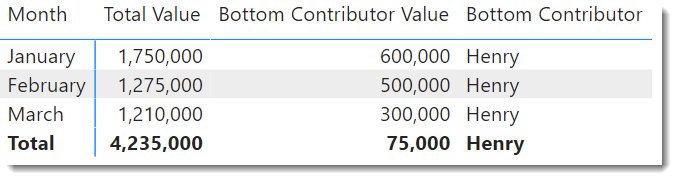cancel
Showing results for
Did you mean:Frequent Visitor

## How to find least contributors to a pool overtime.

Hi there,

We have a pool with several contributors in it. The total sum of the pool value has descreased overtime. How can I find which contributor(s) had the largest drop of the pool value overtime ?

Below is an example on how our data looks like, and it runs over two years and have 5,000 contributors. The date column is a full date column the is hierarchied.

i.e

In Jan we know the pool value was 1,750,000, in Mar 1,210,000. How to find which contributor is the most responsible of this reduced value ? Thanks 😁

 Contributor Date Value of contributor in pool Bob Jan 2022 1,000,000 Feb 2022 500,000 Mar 2022 860,000 Steve Jan 2022 600,000 Feb 2022 700,000 Mar 2022 300,000 Henry Jan 2022 150,000 Feb 2022 75,000 Mar 2022 50,000

1 ACCEPTED SOLUTIONSuper User

You could do something like:

``````Bottom Contributor Value =
CALCULATE(
[Total Value],
FILTER(
TableName,
RANKX(
TableName,
[Total Value],,
ASC,
Dense
) = 2
)
)

Bottom Contributor =
CONCATENATEX(
FILTER(
TableName,
RANKX(
TableName,
[Total Value],,
ASC,
Dense
) = 1
),
TableName[Contributor]
)
``````

Did my answers help arrive at a solution? Give it a kudos by clicking the Thumbs Up!

Proud to be a Super User!

2 REPLIES 2Super User

You could do something like:

``````Bottom Contributor Value =
CALCULATE(
[Total Value],
FILTER(
TableName,
RANKX(
TableName,
[Total Value],,
ASC,
Dense
) = 2
)
)

Bottom Contributor =
CONCATENATEX(
FILTER(
TableName,
RANKX(
TableName,
[Total Value],,
ASC,
Dense
) = 1
),
TableName[Contributor]
)
``````

Did my answers help arrive at a solution? Give it a kudos by clicking the Thumbs Up!

Proud to be a Super User!Super UserDid my answers help arrive at a solution? Give it a kudos by clicking the Thumbs Up!

Proud to be a Super User!